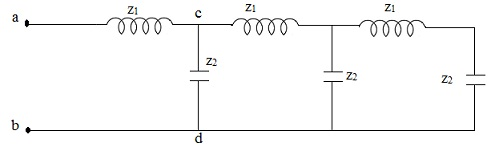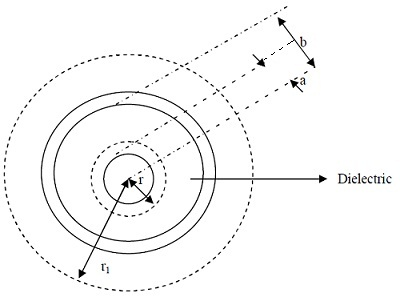#### Transmission lines, Physics tutorial

Transmission lines:

The transmission line is system of material boundaries that forms continuous path which can direct transmission of electromagnetic energy from power station to other stations or for transmitting energy from one point to another. Transmission line is uniform if there is no change in cross sectional geometry. Wavelengths on transmission line are compatible with size at gigahertz frequencies and capacitances and inductances are very small.

Change in potential difference per unit length i.e.

ΔV/ΔL = -LdI/dt = -j(wL + R)I

Or in limit of ΔL becoming dl

dV/dl = -(jwL + R)I

Where L is self inductance because of magnetic field around conductor V and R, is resistance of conductor. Change in current flowing in line is provided by:

dI/Dl = cdV/dt - (jwc + G)V

Where C is capacitance per unit length (formed due to finite distance between conductors of line) and G is conductance per unit length (whose existence is because of dielectric losses of dielectric medium in between conductors). If we suppose that conductors have zero resistance and that they are divided by perfect insulator (in which case transmission line becomes lossless), equations which are basic equations of transmission line becomes,

dV/dl = -LdI/dt

dI/dl = -CdV/dt

Differentiating equations with l and t respectively we have

d2V/dl2 = -L(d2I/dldt)

d2I/dtdx = -Cd2V/dt2

(1/LC)(d2V/dl2) = d2V/dt2

(1/LC)(d2I/dl2) = d2I/dt2

Equations are familiar wave equations of voltage and current respectively mean that both voltage and current propagate as waves along transmission lines. Velocity of propagation is V = 1/√LC. It can be illustrated that characteristic impedance of the transmission line z0 = √LC.Parallel wire and coaxial cable transmission line:

Two major examples of transmission lines are (i) parallel wire and (ii) coaxial cable.

To get propagation velocity, V and characteristics impedance of any transmission line inductance per unit length, L and capacitance per unit length should be determined.

a) Parallel wire transmission lines: Recall that electric field E of conductor is given by Gauss law for electric as,

E = λ/(πrε0εr = q/(2πrε0εrl))

λ(linear charge density) = q/l, for conductor in the medium of relative permittivity (dielectric constant), εr. For two wires, each of radius x and separated by distance y.

E = E1 + E2 = q/2πrε0εrl + q/2πrε0εrl = q/πrε0εrl

Capacitance of pair is obtained from

C = q/V = q/x2yEdr = [q/(q/(πε0εrlx2y(dr/k)))]

Giving

C/l = πε0εr/ln(2y/x) i.e. capacitance per unit length. Inductance per unit length is attained as follows: magnetic flux of two conductors (whose shape are approximately cylindrical),

ΦB = 2lx2y-xμ0μrIdr/2πr ≈ [μ0μrIln(2y/x)/π]

But self inductance, L = ΦB/i = [μ0μrIln(2y/x)/π]

Therefore velocity of propagation, V = 1/√LC = 1/√ε0εrμ0μr as expected. Characteristics impedance,

z0 = √L/C = (μ0μr/π2ε0εr)1/2ln(2y/x)

b) Coaxial cable transmission line: the capacitance for coaxial cable is obtained following procedure utilized for the parallel wire except that ∫Edr is from a to x and b to y, a being radius of central conductor and b, distance between centres of both conductors. Capacitance per unit length is therefore

C/l = 2πε0εr/ln(y/x)Inductance of coaxial cable is attained as follows: flux of magnetic field, ΦB, through closed circuit formed by joining conductors at the end of section of cable of length, l, is lxy0μrI/2πr)dr = (μ0μr/2π)Illn(y/x)

Self inductance, L = ΦB/I = L = (μ0μr/2π)ln(y/x)

Velocity of propagation, V = 1/√LC

Substituting V = 1/√ε0εrμ0μr, and characteristics impedance,

z0 = √L/C(μ0μr/4π2ε0εr)1/2 lny/x

Equivalent circuit:

In Figure 1 above is illustrated two infinitely long lines carrying equal and opposite current. Part between point X and Y is subsection which comprises of impedance z =R+ jωL and admittance y= G+ jωC. Resistance, R and inductance, L are due to length and diameter of conductors while capacitance exists by virtue of close separation of conductors. Conductors being separated by the imperfect insulator or dielectric necessitate leakage of current. This signifies shunt conductance that together with shunt capacitance provides admittance. 2 conductors can therefore be represented in which case resistance, R and conductance, G are negligible. Each subsection is the equivalent circuit and infinitely loss line is considered as cascade of infinite number of such circuits.

Tutorsglobe: A way to secure high grade in your curriculum (Online Tutoring)